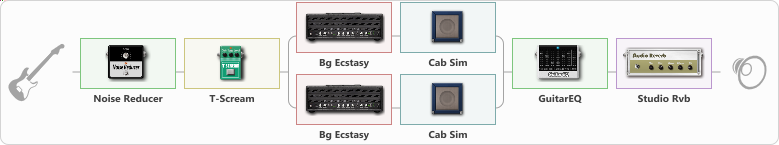# Vallran

Discussion in 'ToneLib-GFX presets' started by Vallran, Dec 31, 2022.

1. Vallran

Preset name: Rammstein Zeit

Effects chain:Effect: "Noise Reducer" (Dynamics / Filter), active - "yes"
{
"Sens" = 66
"Mode" = Soft
}

Effect: "T-Scream" (Overdrive / Distortion), active - "yes"
{
"Drive" = 17
"Tone" = 65
"Level" = 100
}

Effect: "Splitter" (Dynamics / Filter)
{
"A-Bypass" = Off
"A-Pan" = -25
"A-Level" = 55
"B-Bypass" = Off
"B-Pan" = 25
"B-Level" = 55
"Width" = 0

'A' branch:
{

Effect: "Bg Ecstasy" (Amp simulators), active - "yes"
{
"Gain" = 38
"Bass" = 72
"Middle" = 89
"Treble" = 100
"Presence" = 100
"Master" = 100
"Level (dB)" = 0
}

Effect: "Cab Sim" (Cabinets), active - "yes"
{
"Model" = 2x12" Silver Bell
"Level (dB)" = 2
}
}
'B' branch:
{

Effect: "Bg Ecstasy" (Amp simulators), active - "yes"
{
"Gain" = 38
"Bass" = 71
"Middle" = 89
"Treble" = 100
"Presence" = 100
"Master" = 100
"Level (dB)" = 0
}

Effect: "Cab Sim" (Cabinets), active - "yes"
{
"Model" = 2x12" Silver Bell
"Level (dB)" = 2
}
}
}

Effect: "GuitarEQ" (Dynamics / Filter), active - "yes"
{
"160 Hz" = 0
"400 Hz" = -1
"800 Hz" = 3
"1.6 kHz" = 3
"3.2 kHz" = 1
"6.4 kHz" = 0
"12 kHz" = -1
"Level (dB)" = 0
}

Effect: "Studio Rvb" (Reverberation), active - "yes"
{
"Time" = 5.1
"PreDelay" = 7
"LoDamp" = 10
"HiDamp" = 12
"Mix" = 42
}

Note: You will need to download and install the ToneLib-GFX software to use the preset.

File size:
860 bytes
Views:
3,444